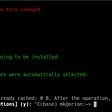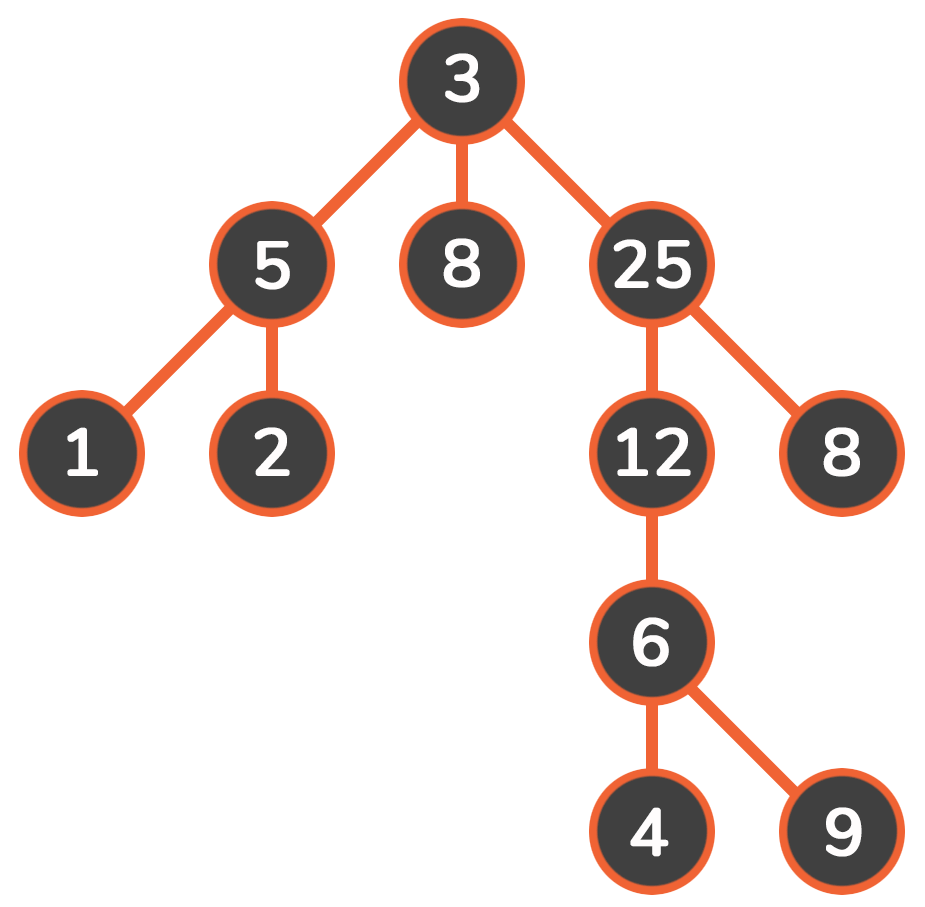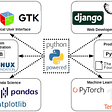# Thinking Recursively In Python

Recursion is such a funny word. Google’s definition of recursion: the repeated application of a recursive procedure or definition. Didn’t Google learn in school that you never define a word by using the word itself? Ironically, that’s what “recursion” is: a function that repeats by calling itself. In this post, my goal is to explain recursion in Python programming in a way that (hopefully) makes sense to someone unfamiliar with the term.

Last week I described how I was humbled during my mock technical interview and decided to strengthen my skills by practicing Python. I described how I had solved the FizzBuzz problem and then explained line-by-line a more streamlined, elegant solution. This week I turned my attention to recursion. In theory, recursion in Python makes sense. For me, though, theory and reality are not always a match. Here are three small challenges that I wrote worked on using recursion: factorial, harmonic sum, and the Fibonacci sequence.

I started “easy” by writing a function to calculate the factorial of a number. In my non-recursive version: call the function, set the result equal to 1 (not 0 because there is multiplication involved and zeros don’t play well here), iterate through the numbers 1 to the n+1 (we can’t multiply by 0 and want to loop through n times), then multiple the result by the new number.

`def nonrec_factorial(n):    result = 1    for i in range(1,n+1):        result = result * i    return result`

Using recursion, I call the function while still in the function as a way to iterate through the numbers. Here is the new code snippet:

`def factorial(n):    while n != 0:        if n == 1:            return 1        return n * factorial(n-1)`

If n is 1 the return is 1 and if n is 0 there is no computation. However, if n is 3, let’s step through the code:

1. We set n == 3, so move to line 5

2. The return is 3 (the current n) times a new call to factorial

3. Start at the top again, with n == 2 this time because 3–1=2

4. Since n is not equal to 1, move to line 5 with n == 2

5. The return is 2 (the current n) times a new call to factorial

6. Start at th top again, with n== 1 because 2–1=1

7. The return is 1

8. The return is 1 times 2 times 3 for a result of 6.

Visually, it looks like this: 3! -> 3 * 2! -> 3 * 2 * 1! -> 3 * 2 * 1 = 6

Next up is finding the harmonic sum for any number n; this one is a bit more fun.

`def sumHarmonics(n):    if n == 0:        return 0    else:        return ( 1/n + sumHarmonics (n — 1))`

Let’s say n =5, the return is:

-> ⅕ + sumHarmonics(4)

-> ⅕ + ¼ + sumHarmonics(3)

> ⅕ + ¼ + ⅓ + sumHarmonics(2)

-> ⅕ + ¼ + ⅓ + ½ + 1 + sumHarmonics(0)

-> ⅕ + ¼ + ⅓ + ½ + 1 = 2.28333

Finally, let’s tackle the Fibonacci sequence using recursion. In the first two series, we specified the number of times we wanted the series to be iterated over and the calculation was done counting down from that specific number. However, with the Fibonacci sequence, the numbers are already known (0,1,1,2,3,5,8,13,21,…) and n indicates how far along the sequence we want to find the sum — to the 5th number, to the 11th number or even the 100th number. This recursive code is a little more tricky to follow:

`def fib(n):    if n == 0:        return 0    elif n == 1:        return 1    return (fib(n-1) + fib(n-2))`

Let’s say we want to find the 4th term of the sequence (the answer is 3) and this is how the code would run:

`                                Fib(4)                           /              \                        Fib(3)    +     Fib(2)                       /      \        /      \                   Fib(2) + Fib(1) + Fib(1) + Fib(0)                  /      \              Fib(1) + Fib(0)`

Where Fib(0) = 0 ( the zero term of the sequence is 0) and Fib(1) = 1 (the first term of the sequence is 1.)

1+0+1+1+0 = 3

Fun fact, in honor of Leonard Bonacci (aka Fibonacci ) November 23 is National Fibonacci Day. 1123 — the first four numbers of the series. And if you are interested in even more on this particular series of numbers, check out this link about the sequence and spiral shells.

--

--

--

## More from Annika Noren

Love podcasts or audiobooks? Learn on the go with our new app.

## Essential Linux Command Line Tips and Tricks## STATIC PODS## Static library vs Shared library## Beginners for programing should learn from more experienced people. -Learning attitude -## Guild sessions highlight for 2020## Developer Experience: The Details Matter## Audio processing in Python with Feature Extraction for machine learning## Depth first search in Python## Chapter 2: 11 questions on how to read/write files in Pandas. [Pandas Tutorials QnA Approach]